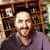# Auto Calculation works in Connect and the Survey123 App but not in the Web Broswer version of the form

680
4
04-23-2021 05:53 AMNew Contributor II

I have a form that is set up to track hours worked on specific projects.  There are two questions asking for time, one asking for a sign-in time, one asking for a sign-out time. Both are formatted as a dateTime.  I have a follow-up question that is read-only and has a calculation attached to it to display the total hours worked between sign-in and sign-out minus 30 minutes for lunch.  The calculation for that field is:

(decimal-time(\${time_out}) - decimal-time(\${time_in})) * 24-.5

This calculation works flawlessly in both Survery123 Connect and the ArcGIS Survey123 app however if I were to open the survey in a web browser, the hours worked question does not populate.  I have a few other areas in my form that have calculations to auto-populate responses and those work fine in both the web version as well as both app versions but no other calculations that handle time specifically.

Tags (3)
1 Solution

Accepted SolutionsbyEsri Contributor

Hi @EOCSupport ,

Could you try replace the decimal-time function with decimal-date-time function as decimal-time is not working well on the web app, i.e.:

if(round((decimal-date-time(\${time_out}) - decimal-date-time(\${time_in})) * 24-.5,2)<4,round((decimal-date-time(\${time_out}) - decimal-date-time(\${time_in})) * 24,2),round((decimal-date-time(\${time_out}) - decimal-date-time(\${time_in})) * 24-.5,2))

Thanks,

Jody Zheng Liu

4 RepliesbyEsri Notable Contributor

Hi @EOCSupport,

What version of Connect was the survey published with? Can you please provide a copy of your xlsx file so we can take a closer look and invesitgate further what causes the calculation to not update?

Regards,

Phil.New Contributor II

Apologies for the delay, the version of connect is 3.12.232 and I have attached a version of the xlsx form.  When the user is signing out, it is total_hours_worked that is not calculating via the web version of Survey123. We have been having our users only input via the app as a work around.byEsri Contributor

Hi @EOCSupport ,

Could you try replace the decimal-time function with decimal-date-time function as decimal-time is not working well on the web app, i.e.:

if(round((decimal-date-time(\${time_out}) - decimal-date-time(\${time_in})) * 24-.5,2)<4,round((decimal-date-time(\${time_out}) - decimal-date-time(\${time_in})) * 24,2),round((decimal-date-time(\${time_out}) - decimal-date-time(\${time_in})) * 24-.5,2))

Thanks,

Jody Zheng LiuNew Contributor II

That did the trick, thank you so much!